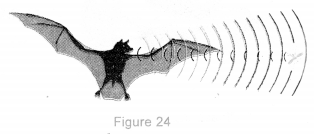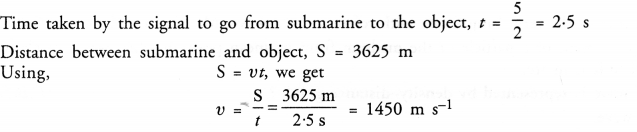# NCERT Solutions for Class 9 Science Chapter 12 Sound

## NCERT Solutions for Class 9 Science Chapter 12 Sound

These Solutions are part of NCERT Solutions for Class 9 Science. Here we have given NCERT Solutions for Class 9 Science Chapter 12 Sound. LearnInsta.com provides you the Free PDF download of NCERT Solutions for Class 9 Science (Physics) Chapter 12 – Sound solved by Expert Teachers as per NCERT (CBSE) Book guidelines. All Chapter 12 – Sound Exercise Questions with Solutions to help you to revise complete Syllabus and Score More marks.

More Resources

NCERT TEXT BOOK QUESTIONS

IN TEXT QUESTIONS

Question 1.
Explain how sound is produced by your school bell.

Or

Sound is produced when your school bell is struck with a hammer. Why ? (CBSE 2011)
When school bell is struck by a hammer, it starts vibrating. Since the vibrating bodies produce sound, so the vibrating school bell produces the sound.

Question 2.
Why are sound waves called mechanical waves ? (CBSE 2012)
Sound waves are characterised by the motion of particles of a medium. Hence sound waves are called mechanical waves.

Question 3.
Suppose you and your friend are on the moon. Will you be able to hear any sound produced by your friend ?
Sound waves need material medium like air to move from one place to another place. Since there is no air on the moon, so sound cannot travel from one place to another place. Hence, we cannot hear sound on the moon.

Question 4.
Which wave property determines
(a) loudness,
(b) pitch ? (CBSE 2011, 2012)
(a) Amplitude of the wave determines loudness.
(b) Frequency of the wave determines pitch.

Question 5.
Guess which sound has a higher pitch : guitar or car horn ?
Guitar, because frequency of sound produced by guitar is higher than the sound produced by car horn.

Question 6.
What are wavelength, frequency, time period and amplitude of a sound wave ? (CBSE 2012)
Wavelength (or length of a wave): The distance between two successive regions of high pressure or high density {or compressions) or the distance between two successive regions of low pressure or low density (or rarefactions) is known as wavelength of a sound wave. It is denoted by λ (read as lambda).
In S.I., unit of wavelength is metre (m).
Frequency: The number of compressions or rarefactions crossing a point per unit time is known as the frequency of a sound wave. It is denoted by μ (read as Neu). In S.I., unit of frequency is hertz (Hz).
1 hertz = one oscillation completed by a vibrating body or a vibrating particle in one second.
Time period: Time taken by two consecutive compressions or rarefactions to cross a fixed point. Amplitude. The maximum displacement of a vibrating body from its rest position or mean position.

Question 7.
How are the wavelength and frequency of a sound wave related to its speed ? (CBSE 2011, 2012)
V = vλ.

Question 8.
Calculate the wavelength of a sound wave whose frequency is 200 Hz and speed is 440 m/s in a given medium.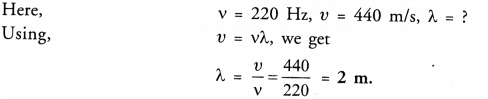Question 9.
A person is listening to a tone of 500 Hz sitting at a distance of 450 m from the source of sound. What is the time interval between successive compressions from the source ?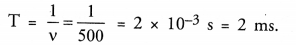Question 10.
Distinguish between loudness and intensity of sound.

 Loudness Intensity of a sound 1.Loudness is a subjective quantity. It depends upon the sensitivity of the human ear. A sound may be loud for a person but the same sound may be feeble for another person who is hard of hearing even when both are sitting at the same distance from the source of sound. Intensity of a sound is an objective physical quantity. It does not depend on the sensitivity of a human ear. 2. Loudness cannot be measured as a physical quantity because it is just sensation which can be felt only. Intensity of a sound can be measured as a physical quantity.

Question 11.
In which of the three media, air, water or iron, does sound travel the fastest at a particular temperature ?
Sound travels the fastest in iron.

Question 12.
An echo returned in 3 s. What is the distance of the reflecting surface from the source, given that the speed of sound is 342 m s-1 ? (CBSE 2011)
Time taken by sound to travel from the source to the reflecting surface, t = 3/2 = 1.5 s
Speed, v = 342 m s
Distance of reflecting surface from the source, S = vt = 342 x 1.5 = 513 m.

Question 13.
Why are the ceilings of concert halls curved ? (CBSE 2011, 2012)
So that the sound after reflection from the ceiling reaches all the corners of the hall.

Question 14.
What is the audible range of the average human ear ? (CBSE, 2011, 2012, 2015)
20 Hz to 20,000 Hz.

Question 15.
What is the range of frequencies associated with :
(a) infra sound ?
(b) ultra sound ? (CBSE 2011, 2013)
(a) Frequencies less than 20 Hz and greater than zero.
(b) Frequencies greater than 20,000 Hz and equal to 107 Hz.

Question 16.
A submarine emits a sonar pulse, which returns from an underwater cliff in 1.02 s. If the speed of sound in salt water is 1531 m/s, how far away is the cliff ?
Time taken by the pulse to go from submarine to the cliff, t = 1.02/2 =0.51 s
Speed of sound, v = 1531 m/s
Distance of cliff from the submarine, S = vt = 1531 x 0.51 = 780.81 m.

NCERT CHAPTER END EXERCISE

Question 1.
What is sound and how is it produced ? (CBSE2012)
Sound is a form of energy which produces the sensation of hearing in our ears. Sound is produced by forcing an object to vibrate. In other words, sound is produced by a vibrating object.

Question 2.
Why is sound wave called a longitudinal wave ? (CBSE 2012)
When sound waves travel in medium, the particles of the medium vibrate about their equilibrium positions along the direction of the propagation of the waves.

Question 3.
Which characteristic of the sound helps you to identify your friend by his voice while sitting with others in a dark room ? (CBSE 2011)
Timber or quality of sound.

Question 4.
Flash and thunder are produced simultaneously. But thunder is heard a few seconds after the flash is seen. Why ?

Or

There is some time interval between observing a flash and hearing a thunder. Explain. (CBSE 2013)
Thunder is heared after some time interval the flash is seen because speed of sound is less than the speed of light.

Question 5.
A person has a hearing range of 20 Hz to 20 kHz. What are the typical wavelengths of sound waves in air corresponding to these two frequencies ?
Take the speed of sound.in air as 344 m s-1.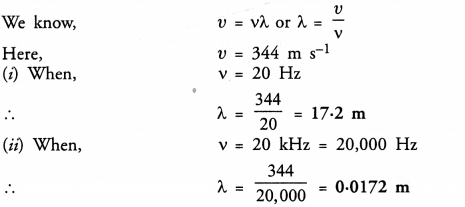Question 6.
Two children are at opposite ends of an aluminium rod. One strikes the end of the rod with a stone. Find the ratio of times taken by the sound waves in air and in the aluminium to reach the second child. (Speed of sound in aluminium is 6420 m s-1 and in air is 346 m s-1). (CBSE 2013)
Let l = length of the rod
Time taken by sound to travel distance / in aluminium rod,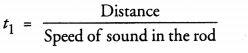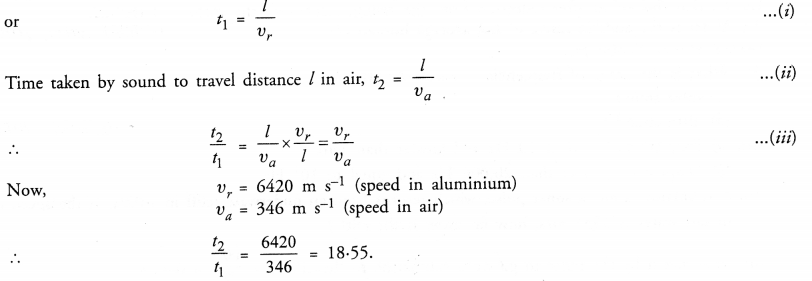Question 7.
The frequency of a source of sound is 100 Hz. How many times does it vibrate in a minute ?
(CBSE 2011, 2012)
Frequency of source = 100 Hz.
Number of times the source of sound vibrates in 1 s = 100
Number of times the source vibrates in a minute or 60 s = 100 x 60 = 6000.

Question 8.
Does sound follow the same laws of reflection as light does ? Explain.
Yes. Sound waves are reflected just like light waves.

Question 9.
When a sound is reflected from a distant object, an echo is produced. Let the distance of the reflecting surface and the source of sound production remains the same. Do you hear echo sound on a hotter day ?
Let d = distance between the reflecting surface and the source of sound
v = speed of sound in air.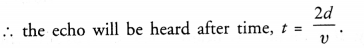On a hotter day, speed of sound increases with increase in temperature. Hence, the time after which echo is heard decreases. If the time taken by the reflected sound is less than 0-1 s after the production of original sound, then echo is not heard. However, if this time is greater than 0-1 s, then echo will be heard.

Question 10.
Give two practical applications of multiple reflection of sound waves. (CBSE 2011, 2012)

1. Megaphone
2. Hearing aid.

Question 11.
A stone is dropped from the top of a tower 500 m high into a pond,of water at the base of the tower. When is the splash heard at the top ? Given g = 10 m s-2 and speed of sound = 340 m s-1 .
(CBSE 2011, 2012)
Time, after which splash is heard = time taken by the stone to reach the surface of water in a pond + time taken by the sound of splash to reach the top of tower.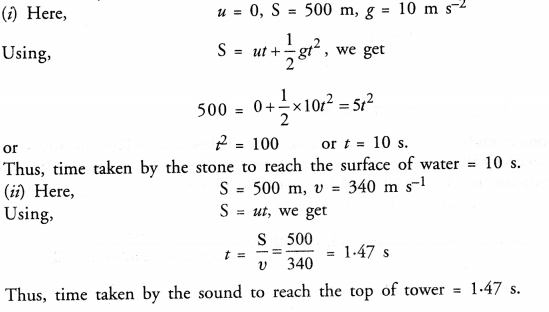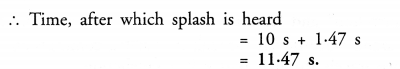Question 12.
A sound wave travels at a speed of 399 m s-1. If its wavelength is 1.5 m, what is the frequency of the wave ? Will it be audible ?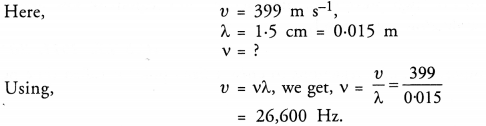Since audible range of frequencies is 20 Hz to 20,000 Hz. Hence, the given frequency will not be audible.

Question 13.
What is reverberation ? How can it be reduced ? (CBSE 2012, 2014)
The phenomenon of prolongation of original sound due to the multiple reflection of sound waves even after the source of sound stops producing sound is called reverberation.
Reverberation can be reduced by covering the roof and walls of a hall by sound absorbing materials.

Question 14.
What is loudness of sound ? What factors does it depend on ? (CBSE 2011)
Loudness of a sound is a subjective quantity which causes unpleasant effect in our ear.
Loudness depends upon the amplitude of the vibrating body and the sensitivity of human ear.

Question 15.
Explain how bats use ultrasound to catch a prey. (CBSE 2011, 2012)

Or

Bats have no eyes, yet they can ascertain distances. (CBSE 2013)